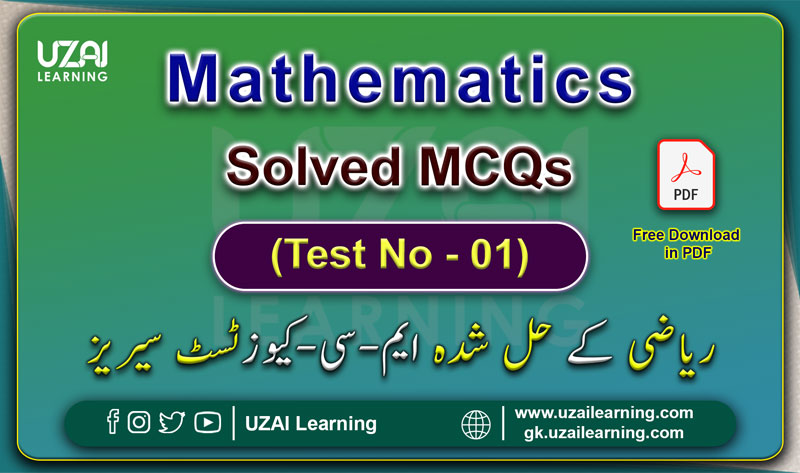# Mathematics Solved MCQs Mock Test No-01, For All Tests Preparation, Prepared by UZAI LearningMathematics Solved MCQs Mock Test No-01, Prepared by UZAI Learning

Mathematics

#### Mock Test Solved MCQs

(Test No - 01)

Mathematics Daily Dose Solved MCQs Test Series for all types of test preparation for example FIA, IB, FBR, ANF, ASF, NTS, PTS, OTS, CTS, FPSC, PPSC, KPPSC, BPSC, SPSC, and other government and private sector test organization. Candidates who have applied to above mentioned organization or else.

You can prepare yourself for written tests and interviews and also free Download all notes, books, and other preparation material for tests in PDF MCQs and Questions Answers Base Information for  FIA, ASF, IB, FBR MCQs, and Test Preparation for All Test Preparation 2023-24.

MCQs Questions and Answers, General Knowledge, Everyday Science, Pak Study, English, Current Affairs, Computer, Islamiyat and Mathematics Up-to-Date Syllabus 2023-24 for FIA, ASF, IB, ANF and other Test preparation of Government and Private Organizations.

پی ڈی ایف میں ڈاونلوڈ کرنے کے لئے نیچے👇 دئیے گئے لنک پر کلک کریں

1. In a certain class the ratio of men to women is 3:5. If the class has 24 people in it, how many are women?

A. 9
B. 12
C. 15
D. 18

2. A lorry covers a distance of 100 km in 2 hours 30 minutes. A car runs with a speed of 55 km/hour. Determine the ratio of their speeds. ​

A. 8:11
B. 20:11
C. 4:11
D. None of These

3. a number exceeds another number by 5. The sum of the numbers is 19. find the smaller number?

A. 5
B. 6
C. 7
D. 12

4. If Arif can read 39 pages of a book in half an hour. How long will It take her to finish a book that is 1287 pages?

A. 16 Hours
B. 16.5 Hours
C. 17 Hours
D. 15.5 Hours

5. If Ahmed purchases a Watch for Rs 600 and pays 15% sales tax, the total amount spent on purchase is?

A. 660
B. 670
C. 680
D. 690
E. 700

6. What is the Arithmetic Mean of the following data 3,6,9,12,15.

A. 7
B. 9
C. 12
D. 8

7. A student can solve q questions in 1/h hour. At this rate how many questions can she solve in q hours?

A. hq
B. hq2
C. 1/hq
D. h/q

8. complete the series: 4.8.9.13.14.18. _______?

A. 22
B. 32
C. 11
D. 19

9. A person purchases 90 clocks and sells 40 clocks at a gain of 10% and 50 clocks at a gain of 20%. If he sold all of them at a uniform profit of 15%, then he would have got Rs. 40 less. The cost price of each clock is: _________?

A. Rs.50
B. Rs.60
C. Rs.80
D. Rs.90

10. The cash difference between the selling prices of an article at a profit of 4% and 6% is Rs. 3. The ratio of the two selling prices is: __________?

A. 51:52
B. 52:53
C. 51:53
D. 52:55

11. If 5% more is gained by selling an article for Rs. 350 than by selling it for Rs. 340, the cost of the article is: ________?

A. Rs. 50
B. Rs. 160
C. Rs. 200
D. Rs. 225

12. A man buys an article for 10% less than its value and sells it for 10% more than its value. His gain or loss percent is: ________?

A. no profit, no loss
B. 20% profit
C. less than 20% profit
D. more than 20% profit

13. A manufacturer sells a pair of glasses to a wholesale dealer at a profit of 18%. The wholesaler sells the same to retailer at a profit of 20%. The retailer in turn sells them to a customer for Rs. 30.09, there by earning a profit of 25%. The cost price for the manufacturer is: ________?

A. Rs. 15
B. Rs. 16
C. Rs. 17
D. Rs. 18

14. A sells a bicycle to B at a profit of 20%. B sells it to C at a profit of 25%. If C pays Rs. 225 for it, the cost price of the bicycle for A is: ________?

A. Rs. 110
B. Rs. 120
C. Rs. 125
D. Rs. 150

15. A fair price shopkeeper takes 10% profit on his goods. He lost 20% goods during theft. His loss percent is: ________?

A. 8
B. 10
C. 11
D. 12

16. By mixing two brands of tea and selling the mixture at the rate of Rs. 177 per kg. a shopkeeper makes a profit of 18%. If to every 2 kg of one brand costing Rs. 200 per kg, 3 kg of the other brand is added, then how much per kg does the other brand cost?

A. Rs. 110
B. Rs. 120
C. Rs. 140
D. None of these

17. Haroon purchased 30 kg of wheat at the rate of Rs. 11.50 per kg and 20 kg of wheat at the rate of 14.25 per kg. He mixed the two and sold the mixture. Approximately what price per kg should be sell the mixture to make 30% profit?

A. Rs. 14.80
B. Rs. 15.40
C. Rs. 15.60
D. Rs. 16.30
E. Rs. 18.20

18. Which Muslim scientist wrote first book on Algebra?

A. Al-Khwarizmi
B. Ibn Sina
C. Archimedes
D. Euclid

19. What’s the largest known Prime Number?

A. 282,589,933 − 3
B. 282,589,933 − 1
C. 282,589,933 − 5
D. None of these

20. Find two numbers whose sum is 28 and the difference is 4 _____________?

A.  12,16
B.  18,10
C.  15,13
D.  14,12

پی ڈی ایف میں ڈاونلوڈ کرنے کے لئے نیچے👇 دئیے گئے لنک پر کلک کریں